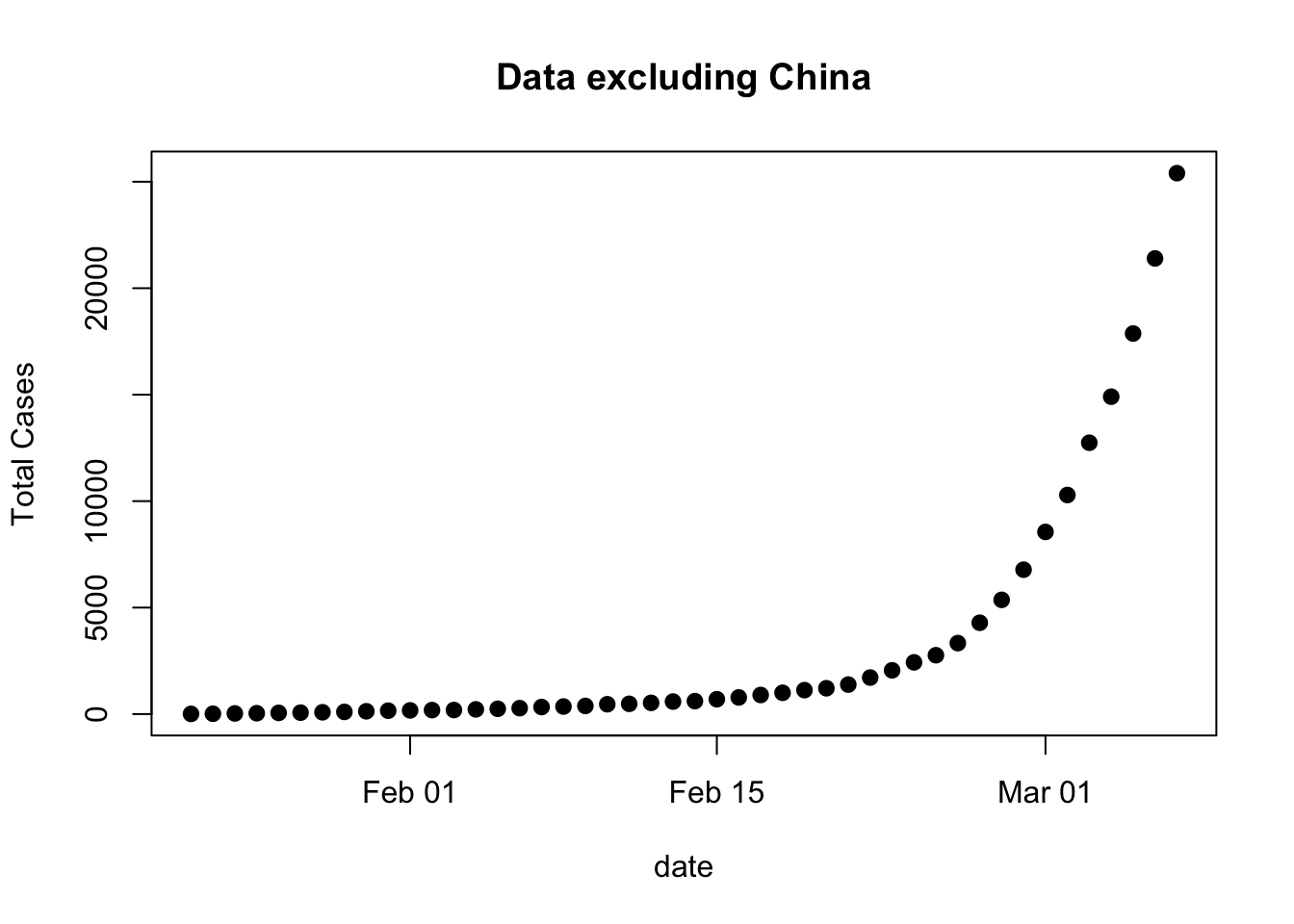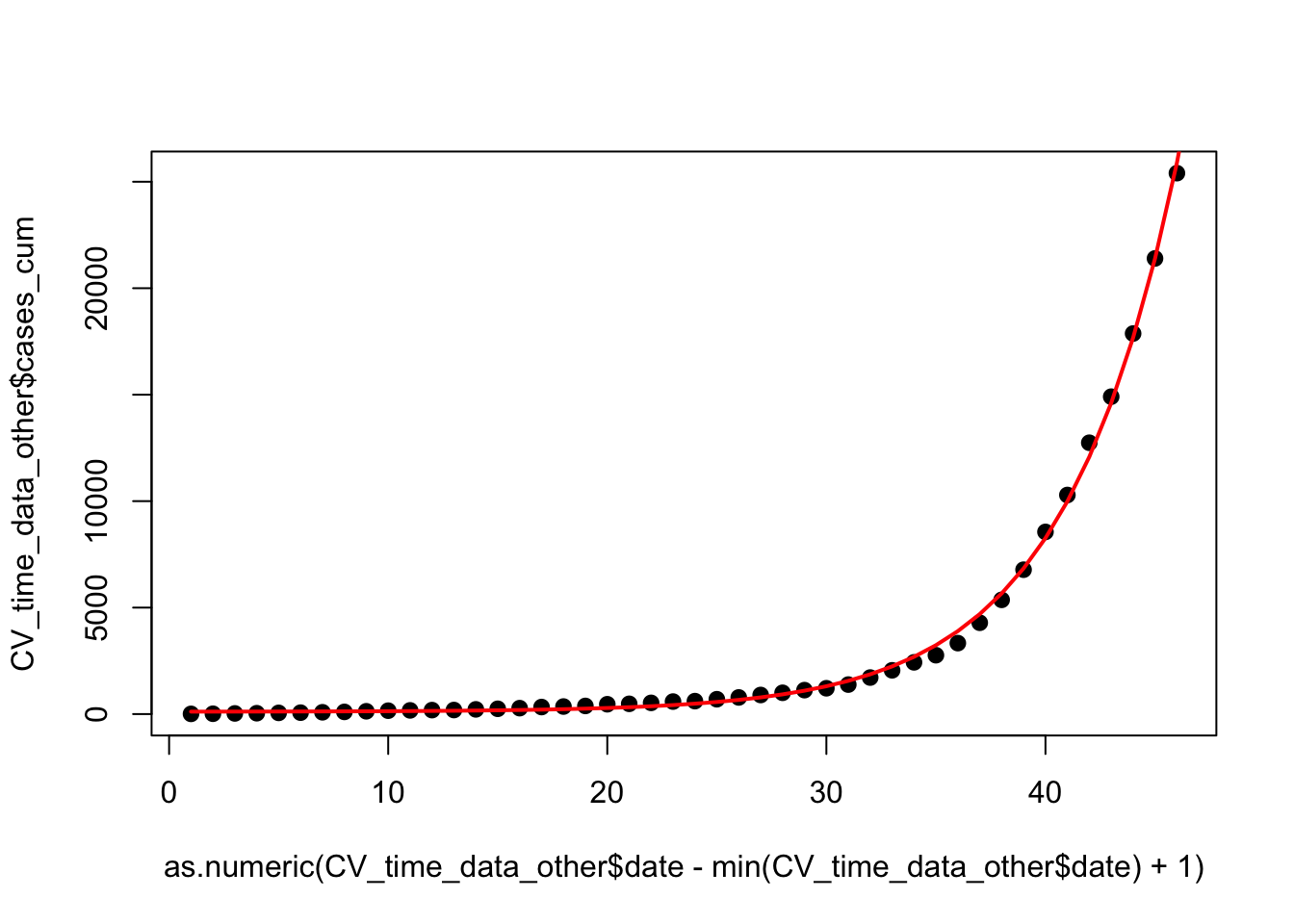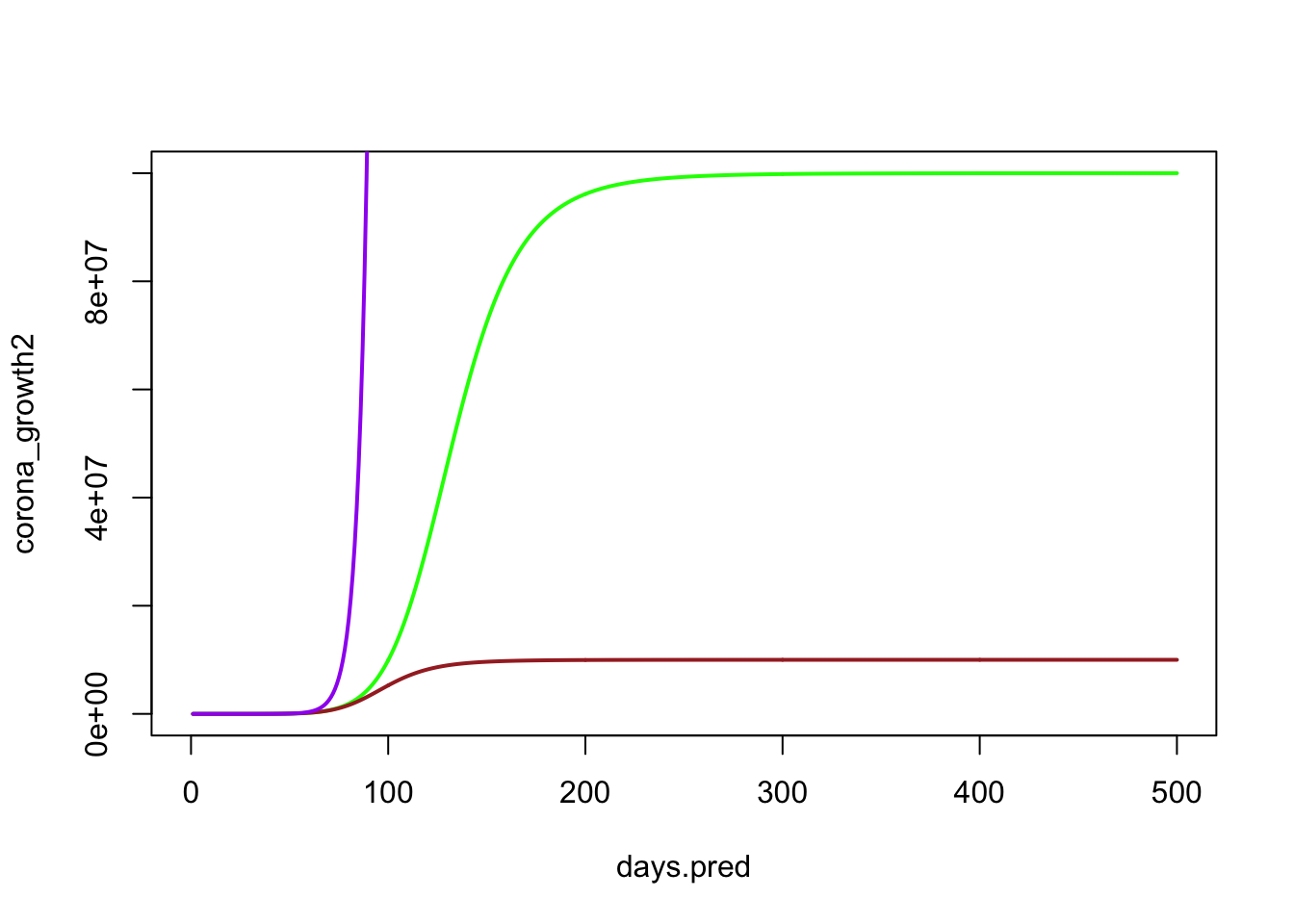#### Analysis to predict the arrival of CoronaVirus to the Galapagos Archipelago

This analysis is based on available data from several sources:

• WorldBank country population data
• PNG tourist visitation data (2017 report)
• Johns Hopkins University CoronaVirus data (package ‘coronavirus’)
• Worldometer.com CoronaVirus numbers for each country

In essence the analysis is based on a simulation in which every day a random group of tourists are selected from each country based on tourist visitation statistics for Galapagos. The proportion of actively infected individuals in that country affects the probability that one of those tourists is infected. Each day that passes the total number of infected individuals in each country increases, and so does the probability that one of those individuals will visit the Galapagos.

### 1) Results and conclusions

#### (updated March 8, 2020):

1. Under the most extreme growth scenario, Galapagos has a 95% probability of being infected by April 10, with a 50% chance of infection by April 2nd

2. Reducing tourist visitation to Galapagos by half has a very small effect of delaying infection (difference of only several days)

3. Assuming a slower growth rate of the virus with an asymptote at 100 million, Galapagos is still highly likely to be infected by April 28 THIS IS AN UPGRADE OF TWO MONTHS FROM PREVIOUS ESTIMATE

4. Additional reduction to an asymptote of only 10 million, yields a similar prediction of 95% chance of infection by May 2nd. THIS IS A SIGNIFICANT UPGRADE OF MORE THAN THREE MONTHS FROM PREVIOUS ESTIMATE

5. Even a best-case scenario in which growth asymptotes at 10 million, tourism is reduced to half, all Chinese tourism is excluded, and the conservative assumption that the proportional infection of each country is estimated using the entire population of each country instead of just the urban population yields a prediction in which there is a 50% chance of infection by April 28.

Simulating different scenarios that vary from extreme growth of the virus to slower, more optimistic projections, still leads to the similar conclusion that the question is likely not if COVID-19 reaches Galapagos, but when it does. In addition, these estimates are likely conservative due to the much larger potential for undocumented or diagnosed cases as has been suggested. However, the time until the virus reaches Galapagos is largely dependent on the spread of the virus, and much is still unknown about this. I am not an epidemiologist, and though my models of the growth of the virus might not be fully accurate, the fact that several different growth functions led to similar conclusions is intriguing. I think that the simplicity of this analysis coupled with the results should encourage the Galapagos hospitals and community to prepare. If COVID-19 reaches the Galapagos, the effect would be devastating. I hope that some evidence demonstrating the likely potential for the virus reaching Galapagos is enough to help us take better action to prepare just in case. Assuming the best case scenario is not a risk we should take.

### 2) Data collection and cleaning

The first step is to load all necessary functions and packages:

``````library(tidyverse)
library(lubridate)
library(wbstats) # world_bank_pop
library(coronavirus) # johns hopkins coronavirus data
library(mgcv)``````

The next step is to collect and process the data:

#### WorldBank Population Data:

``````# Extract country codes to rename to normal country names:
country_codes <- wb_cachelist\$countries %>%
select(iso3c, country) %>%
mutate(country_name = country,
country = iso3c) %>%
select(-iso3c)

# Now extract the population and urban population proportion from each country
# (seems like 2017 is the latest available data for WorldBank populations)
country_pop <- filter(world_bank_pop, indicator=="SP.POP.TOTL" | indicator=="SP.URB.TOTL") %>%
select(country,indicator,`2017`) %>%
pivot_wider(names_from = indicator, values_from = `2017`) %>%
mutate(percent_urban = SP.URB.TOTL/SP.POP.TOTL) %>%
left_join(country_codes) %>%
mutate(population = SP.POP.TOTL,
# Since population growth in last three years averages 1.1%, all populations were
# increased by that amount to project the 2019 numbers:
population = population + (population*0.011), # 2017 --> 2018 growth
population = population + (population*0.011), # 2018 --> 2019 growth
population = round(population)) %>%
select(country=country_name, population, percent_urban)

country_pop``````

#### PNG Tourist Visitation Data:

Load data that was manually extracted from the 2017 PNG tourist visitation report:

First extract the data on the number of visitors from each country. Those numbers were manually projected to 2020:

``````gal_visit_country <- read_csv("data/galapagos_visitation_2017.csv") %>%
rename(visitors_2017 = number_2017,
total_visitors_2017 = total)

gal_visit_country``````

Then extract the number of monthly visitors for 2015, 2016, and 2017 to generate a monthly average visitation rate:

``````gal_visit_month <- read_csv("data/galapagos_monthly_visitation_2015_2017.csv")

### Average the proportion of vistors each month and
### calculate the proportion of yearly visitors each day:
gal_monthly_sum <- group_by(gal_visit_month, month) %>%
summarize(mean_num = mean(number)) %>%
ungroup() %>%
mutate(monthly_prop = mean_num/sum(mean_num),
daily_prop = monthly_prop/c(31,28,31,30,31,30,31,31,30,31,30,31),
month=as.factor(month))

gal_monthly_sum``````

#### Worldometers.info Data on Cases by Country:

As of Mar 8

``````#latest_CV_data <- read_csv("data/Feb 26 Coronavirus data.csv")
select(country="Country,Other",total_cases="TotalCases",new_case=NewCases, deaths=TotalDeaths, new_deaths=NewDeaths, total_rec=TotalRecovered, total_critical="Serious,Critical") %>%
mutate(country = recode(country, "S. Korea"="South Korea", "USA"="United States", "UK"="United Kingdom")) %>%
filter(country != "Total:")

latest_CV_data``````
``````### Use non-china growth data to model growth rate differently for other countries: www.worldometer.info/coronavirus/coronavirus-cases
date <- seq.Date(as.Date("2020-01-22"), as.Date("2020-03-07"), by="day")
cases_cum <- c(9,15,30,40,56,66,84,102,131,159,173,186,190,221,248,278,330,354,382,461,
481,526,587,608,697,781,896,999,1124,1212,1385,1715,2055,2429,2764,3332,4288,5364,
6780,8555,10288,12742,14906,17872,21398,25403)
cases <- NA
CV_time_data_other <- tibble(date,cases,cases_cum)
CV_time_data_other\$cases <- c(0,diff(CV_time_data_other\$cases_cum))
plot(cases_cum~date, pch=16, cex=1.2, data=CV_time_data_other, main="Data excluding China", ylab="Total Cases")``````#### Now combine and process the data:

``````### Calculate the total number of cases worldwide:
total_cases <- apply(select(latest_CV_data, -country, -new_case),2,FUN=function(x) sum(x,na.rm=T))

### Connect CV by country data to galapagos country visits data to filter
### only those countries that visit Galapagos:
combined_data <- left_join(gal_visit_country, latest_CV_data)
galap_num_data <- select(combined_data, names(total_cases))

### Now, need to calculate the total number of cases for the "All other" countries row:
total_cases_gal <- apply(galap_num_data,2,FUN=function(x) sum(x,na.rm=T))
other_countries <- total_cases - total_cases_gal
for(i in names(other_countries)){
combined_data[3,i] <- other_countries[i]
}

### Replace any NAs with generated after combining the data sets with zeros:
combined_data_clean <- mutate(combined_data,
total_cases = replace_na(total_cases, 0),
deaths = replace_na(deaths, 0),
new_deaths = replace_na(new_deaths, 0),
total_rec = replace_na(total_rec, 0),
total_critical = replace_na(total_critical, 0))

### now add the country population data:
data_semifin <- left_join(combined_data_clean, country_pop) %>%
mutate(urban_pop = percent_urban*population)

### Again, need to fill in the "All other" countries row with population data:
urban_pop_tot <- sum(data_semifin\$urban_pop, na.rm=T)
# total urban population of the world is 4.2 billion, so the 2509 other
# coronavirus cases come from the total remaining urban population
# (in other words, exclude the population of those already on the list):
total_remaining_urb_pop <- 4200000000 - urban_pop_tot

### do the same for total population of each country:
normal_pop_tot <- sum(data_semifin\$population, na.rm=T)
total_remaining_norm_pop <- 7530000000 - normal_pop_tot

### now put those in the data:
data_semifin[3,"population"] <- total_remaining_norm_pop
data_semifin[3,"urban_pop"] <- total_remaining_urb_pop
data_semifin[3,"percent_urban"] <- total_remaining_urb_pop/total_remaining_norm_pop

### Calculate proportion of infected that each country has:
data_fin <- select(data_semifin, country, gal_visitors = proj_2020,
total_CV_cases=total_cases, cntry_urban_pop=urban_pop,
population) %>%
# adding 1 to all countries to make sure they all have at least s
# some chance of becoming infected.
mutate(total_CV_cases = total_CV_cases+1,
gal_visitors = round(gal_visitors),
total_CV_cases_prop = total_CV_cases/sum(total_CV_cases))

data_fin``````

### 3) Model worldwide growth of CV

The following is a function that can be used to model the growth rate of Coronavirus under different scenarios. The parameters allow you to:

• Set the model type (logistic, exponential, or linear; “type”)
• Set the logistic limit (where the total number of cases levels to; “max_infected”)
• The number of days to predict this growth for (“days2pred”)
• The option to return only the active number of cases each day (rather than cumulative total; “return_active”)
``````growth_model <- function(max_infected=2000000, type="exp", days2pred=c(1:200), CVdata=CVdata.nospike, return_active=F){
#date_values <- seq.Date(as.Date("2020-01-22"), as.Date("2020-03-31"), by="day")
days <- as.numeric(CVdata\$date-min(CVdata\$date))+1
### First estimate starting parameters from linear model:
### Select an approximate theta, since theta must be lower than min(y), and greater than zero
theta.0 <- min(CVdata\$cases_cum) * 0.5
model.0 <- lm(log(cases_cum-theta.0) ~ days, data=CVdata)
alpha.0 <- exp(coef(model.0))
beta.0 <- coef(model.0)
start <- list(alpha = alpha.0, beta = beta.0)
start.thet <- list(alpha = alpha.0, beta = beta.0, theta=theta.0)

days.pred <- days2pred

if(type=="log"){
start <- list(xmid = 5, scal = 1)
nls.model.logist <- nls(cases_cum ~ max_infected/(1+exp((xmid-log(days))/scal)),
data=CVdata, start=start)
#nls.model.logist <- nls(cases_cum ~ SSlogis(log(days), Asym, xmid, scal), CVdata)
#nls.model.logist <- nls(cases_cum ~ max_infected/(1+alpha*exp(beta*days)), data=CVdata, start=start)
corona_pred <- predict(nls.model.logist, list(days=days.pred))
}

if(type=="exp"){
nls.model <- nls(cases_cum ~ alpha * exp(beta*days)-theta, data=CVdata, start=start.thet)
corona_pred <- predict(nls.model, list(days=days.pred))
# nls.model.theta <- nls(cases_cum~ alpha * exp(beta*days)-theta, data=CV_time_data, start=start.thet)
# corona_pred <- predict(nls.model.theta, list(days=days.pred))
}

if(type=="lin"){
lm.model <- lm(cases_cum ~ days, data=CVdata)
corona_pred <- predict(lm.model, list(days=days.pred))
}

### Now calculate the total number of active cases without symptoms (rolling
### total every 15 days) for each prediction: To do that, calculate how many
### new cases there are each day, and from that calculate a moving window
### cumulative sum within 15 days.

number_new_cases <- corona_pred - lag(corona_pred)
number_new_cases <- corona_pred
number_new_cases <- ceiling(number_new_cases)

active_cases <- NULL
for(i in 1:length(number_new_cases)){
# active cases are the sum of the range of cases from
active_cases[i] <- sum(number_new_cases[max(c(i-15,1)):i])
}
if(return_active) return(active_cases) else return(corona_pred)
}``````

### 4) Create a few other essential functions

Different countries have a different proportion of cases, possibly due to differnces in travel habits, or where the virus began in the first place (e.g., China). Assuming these proportions remain the same over time, to figure out how many people are infected within each country at any point in time, just take the total number infected globaly and multiply that by the relative country proportions. Here is a function that does that:

``````projected_CV_by_country <- function(total_infected) {
country_proj <- ceiling(data_fin\$total_CV_cases_prop * total_infected)
names(country_proj) <- data_fin\$country
return(country_proj)
}
# for example:
projected_CV_by_country(10000)``````
``````##        Ecuador  United States      All other United Kingdom        Germany
##              2             35           1440             20             76
##         Canada      Australia      Argentina         France    Switzerland
##              6              7              1             68             26
##          Chile    Netherlands          Spain          Italy          China
##              1             18             48            558           7636
##          Japan        Denmark         Israel    New Zealand         Sweden
##             44              3              3              1             16``````

Now create a function that calculates the total number of individuals arriving daily to Galapagos from each country based on what month it is (since visitation changes from month to month):

``````daily_visitors <- function(month="february"){
daily_prop <- gal_monthly_sum\$daily_prop[gal_monthly_sum\$month==month]
daily_vis <- ceiling(daily_prop*data_fin\$gal_visitors)
names(daily_vis) <- data_fin\$country
return(daily_vis)
}
# for example:
daily_visitors(month="june")``````
``````##        Ecuador  United States      All other United Kingdom        Germany
##            219            203             50             36             29
##         Canada      Australia      Argentina         France    Switzerland
##             26             21             19             13             12
##          Chile    Netherlands          Spain          Italy          China
##             12             12             12             10              7
##          Japan        Denmark         Israel    New Zealand         Sweden
##              5              4              3              3              2``````

This is a function to sample visitors from their respective country populations to create the random draw of potentially selecting a coronavirus infected individual.

• day is what day in the simulation is being selected. A For Loop is used to loop through all the days.
• active_cases is what the growth model outputs (a vector of predicted active cases begining with day one)
• no_china is an option to test what happens if Chinese visition to Galapagos is completely halted to Galapagos
• reduce_visit is an option to see what happens if all tourist visitation to Galapagos is halved
• use_urban is an option to only sample the urban population for coronavirus, assuming that the majority of cases will be in urban centers. Turning this option off will include the entire population of a country in the analysis, making it less likely to randomly select an individual infected with the virus.
``````corona_spread_simu <- function(day=1, active_cases=scenario3, no_china=F, reduce_visit=F, use_urban=T){
# Select the total active for the day:
total_active <- active_cases[day]

# if the total active is less than zero (artifact of the growth model),
# then make total active just 1
if(total_active<0) total_active <- 1

# Calculate the total number of active cases within each country:
total_active_per_country <- projected_CV_by_country(total_active)

# Based on the current day, determine what month it is:
month <- tolower(as.character(month(min(CV_time_data_other\$date)+day, label=T, abbr=F)))

# Calculate daily visitation rate to galapagos based on that month
daily_vis <- daily_visitors(month=month)

# apply options to remove Chinese visitation or halve all visitation:
if(no_china) daily_vis["China"] <- 0
if(reduce_visit) daily_vis <- round(daily_vis *0.5)

# Now is the key... loop through each country to sample visitors for coronavirus:
for(i in 1:nrow(data_fin)){

# Get the population of that country (either urban or total):
if(use_urban==T) pop <- data_fin\$cntry_urban_pop[i]
if(use_urban==F) pop <- data_fin\$population[i]

# Calculate the proportion of that country that is infected:
prop_infect <- total_active_per_country[i]/pop

# Select random numbers from 0 to 1 based on how many visitors there are that day from that country
samp <- runif(daily_vis[i], min=0,max=1)

# if any of those are less than or equal to the proportion infected in that country,
# then someone is infected.
CV_infected <- which(samp <= prop_infect)

# if someone is infected, then exit the loop.
if(length(CV_infected)>0) break #if infected, then break the loop
}
# if someone was infected, return the country name, otherwise return 0.
if(length(CV_infected)>0) return(data_fin\$country[i]) else return(0)
}``````

### 5) Create scenarios for running the simulation:

``````### Example fit:
corona_expon <- growth_model(type="exp", days=c(1:100), CVdata=CV_time_data_other)
plot(CV_time_data_other\$cases_cum~as.numeric(CV_time_data_other\$date-min(CV_time_data_other\$date)+1), pch=16, cex=1.2)
points(corona_expon ~ c(1:100), lwd=2, type="l",col = "red")````````````days.pred <- c(1:500) # predict each scenario across 500 days:

data_to_use <- CV_time_data_other # Data on the growth of CV excluding China
#data_to_use <- CVdata.nospike    # Data on growth including china and removing the spike
#data_to_use <- CV_time_data_new  # Using all the latest data including china

### Scenario 2: Logistic Growth with level off at 100 million people:
scenario2 <- growth_model(max_infected=100000000, type="log", days=days.pred, CVdata=data_to_use, return_active=T)
corona_growth2 <- growth_model(max_infected=100000000, type="log", days=days.pred, CVdata=data_to_use)
plot(corona_growth2 ~ days.pred, lwd=2, type="l",col = "green", xlim=c(0,500), ylim=c(0,100000000))

### Scenario 3: Logistic Growth with level off at 10 million people:
scenario3 <- growth_model(max_infected=10000000, type="log", days=days.pred, CVdata=data_to_use, return_active=T)
corona_growth3 <- growth_model(max_infected=10000000, type="log", days=days.pred, CVdata=data_to_use)
points(corona_growth3 ~ days.pred, lwd=2, type="l",col = "brown")

### Scenario 1: Exponential growth of the virus
scenario1 <- growth_model(type="exp", days=days.pred, CVdata=data_to_use, return_active=T)
corona_growth1 <- growth_model(type="exp", days=days.pred, CVdata=data_to_use)
points(corona_growth1 ~ days.pred, lwd=2, type="l",col = "purple")``````### 5) Run the simulation for each scenario:

Now here is function for running the actual simulation itself (looping through the days):

• scenario is the active cases over time output from the different virus growth scenarios generated above
• title is the title of each plot
• runs is how many times to run the simulation (each time it goes until corona virus reaches Galapagos)
• no_china and reduce_visit are parameters from before to test what happens if tourism changes
• use_urban if true uses only the urban population of a country to sample for corona cases rather than the total
``````run_simulation <- function(scenario=scenario1, title="scenario1", runs=200,
no_china=F, reduce_visit=F, use_urban=T, growth=corona_growth1, leg_place="topleft"){
# create these variables to hold simulation vector results:
time_till_arrival <- NULL
country_from <- NULL

# Setting the number of days to run each simulation round for:
#days_run <- c(1:200)
days_run <- c(1:length(scenario))

for(i in 1:runs){ # run the simulation a certain number of times (each generates a date of arrival)
#print(i)
for(d in days_run){ # within each run, go at least a certain number of days into the future
# apply the simulation function:
reduce_visit=reduce_visit, use_urban=use_urban)
if(infected!=0){ # if someone is infected, then record the county and number of days it took
time_till_arrival <- c(time_till_arrival, d)
country_from <- c(country_from, infected)
break
}

# If the loop gets to the end of the number of days selected
# but still not found someone infected, then set the time_till_arrival to 9999
if(d == max(days_run)){
time_till_arrival <- c(time_till_arrival, 9999)
country_from <- c(country_from, "Unknown")
}
}
}

# convert days of arrival to date format
dates_of_arrival <- min(CV_time_data_other\$date)+time_till_arrival
dates_of_growth <- min(CV_time_data_other\$date)+c(1:length(growth))
names(growth) <- dates_of_growth

# convert dates of arrival into a cumulative probability of arrival:
arrivals <- table(dates_of_arrival)
dates <- as.Date(names(table(dates_of_arrival)))
prob_arrival <- cumsum(arrivals)/max(cumsum(arrivals))*100

# now plot the cumulative probability and label the date
# when 95% probability of arrival has occured:
plot(prob_arrival~dates, type="l",
ylab="Probability of Arrival", xlab="Date", main=title, lwd=2, xlim=as.Date(c("2020-01-22", "2020-07-31")))
#perc_50 <- prob_arrival[which(prob_arrival>50)]
#perc_80 <- prob_arrival[which(prob_arrival>80)]
which_perc_95 <- which(prob_arrival>95)
perc_95 <- prob_arrival[which_perc_95]

which_perc_50 <- which(prob_arrival>50)
perc_50 <- prob_arrival[which_perc_50]

total_at_95 <- growth[names(which_perc_95)]/1000000

#abline(v=as.Date(names(perc_50)))
#abline(v=as.Date(names(perc_80)))
abline(v=as.Date(names(perc_95)), col="red", lwd=2)
abline(v=as.Date(names(perc_50)), col="blue", lwd=2)
#arrows(x0=max(names(prob_arrival)), y0, x1, y1)
#text(names(perc_50), x=as.Date(names(perc_50))-10, y=85)
#text(names(perc_80), x=as.Date(names(perc_80))+10, y=15)
#text(names(perc_95), x=as.Date(names(perc_95))-10, y=45, font=2)
#text("50%", x=as.Date(names(perc_50))-10, y=90)
#text("80%", x=as.Date(names(perc_80))+10, y=20)
#text("95%", x=as.Date(names(perc_95))-10, y=55, font=2)
date_at_95 <- names(perc_95)
if(as.Date(names(perc_95))>as.Date("2040-10-10")) date_at_95 <- NA

date_at_50 <- names(perc_50)
if(as.Date(names(perc_50))>as.Date("2040-10-10")) date_at_50 <- NA

legend(leg_place, lwd=2, col=c("red","blue","white"), c(paste("Date at 95% =", date_at_95),
paste("Date at 50% =", date_at_50),
paste("Cases at 95% =", round(total_at_95,2), "mil")), cex=0.9)
#legend("topleft", lwd=2, col="red", c(paste("Date at 95% =", date_at_95)))

return(prob_arrival)
}``````

Now run the simulations:

``````number_runs <- 400
set.seed(1234)
scen1 <- run_simulation(scenario=scenario1, growth=corona_growth1, title="Exp. growth of CV", runs=number_runs, no_china=F, reduce_visit=F, use_urban=T, leg_place="topright")</``````